U.S. Department of Transportation
1200 New Jersey Avenue, SE
Washington, DC 20590
202-366-4000

Federal Highway Administration Research and Technology
Coordinating, Developing, and Delivering Highway Transportation InnovationsThis report is an archived publication and may contain dated technical, contact, and link information
 Federal Highway Administration > Publications > Research Publications > Hydraulics Publications > 07026 > Bottomless Culvert Scour Study: Phase II Laboratory Report
 Publication Number: FHWA-HRT-07-026 Date: February 2007

# Bottomless Culvert Scour Study: Phase II Laboratory Report

### Chapter 3: Theoretical Background

Experiments show that scour is generally deepest near the corners at the upstream entrance to the culvert. This observation is commonly attributed to the contraction (concentration) of flow near the upstream entrance of the culvert. Figure 4 illustrates the pattern of primary flow near this location, where water that is blocked by the embankments (in the approach to the culvert) is forced through the culvert opening. The vortices and strong turbulence just downstream of the culvert inlet, generated by the contraction of flow and typically called secondary flow, occur in the so-called separation zone. This flow pattern is very similar to the abutment scour phenomenon that researchers have observed for bridge scour.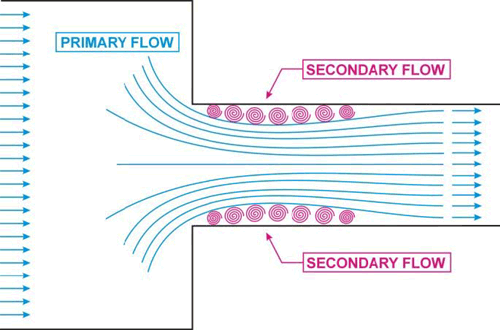Figure 4. Diagram. Flow concentration and separation zone.

Several researchers, including Chang, GKY and Associates, Inc., and Sturm, have suggested that bridge abutment scour can be analyzed as a form of flow distribution scour by incorporating an empirical adjustment factor to account for vorticity and turbulence.(2, 3, 4) The adjustment factor to account for vorticity and turbulence can be derived from laboratory results. These notions were used to formulate the theoretical background for analyzing the culvert scour data. Variables used in the data analysis are illustrated in the following definition sketches for unsubmerged (figures 5 and 6) and submerged (pressure) (figures 7 and 8) flow conditions. The notations in these figures are defined after the last figure.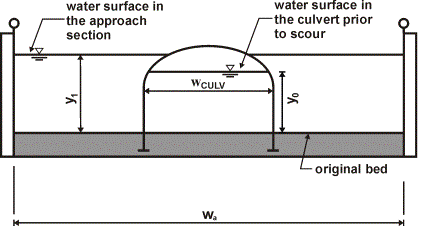Figure 5. Diagram. Definition sketch before scour for unsubmerged flow conditions.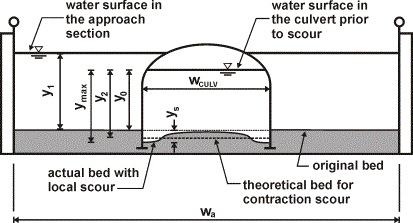Figure 6. Diagram. Definition sketch after scour for unsubmerged flow conditions.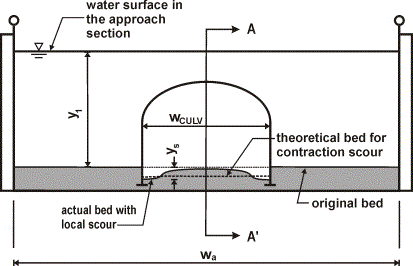Figure 7. Definition sketch after scour for submerged flow conditions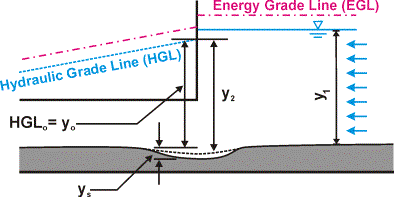Figure 8. Diagram. Side view after scour for submerged flow conditions (Section A-A′ in figure 7).

wCULV
is width of the culvert.
wa
is width of the approach channel.
y1
is water depth in the approach channel at a distance three times wCULV upstream of the culvert entrance.
y0
is water depth at the culvert entrance before scour occurs.
ymax
is maximum water depth in the culvert after scour hole develops.
y2
is equilibrium water depth after scour hole develops.
yS
is maximum depth of scour in the culvert.

#### CLEAR WATER SCOUR

Equation 1 is an expression for the unit discharge for an assumed flow distribution that remains constant as the scour hole develops. If no sediment is being transported into the scour hole, as was the case with all of our experiments, then no sediment can be transported out of the scour hole at equilibrium. In this case, the local velocity must be reduced to the critical incipient motion velocity, Vc, for the sediment size at the equilibrium flow depth, y2. This equation forms the basis for the analysis: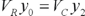(1)

where:

VR
is representative (local) velocity at the entrance of the culvert.
VC
is critical velocity at which incipient sediment motion occurs.

Note that the term on the left side of the equation is the assumed representative unit discharge across the scour hole at the beginning of scour, or qR.

Equation 1 can be rearranged to yield an equilibrium flow depth, y2, once the representative velocity, VR, and the critical incipient motion velocity, VC, have been determined. This equilibrium depth reflects the scour that is attributed to the incoming flow distribution. The next two subsections will illustrate several ways to calculate the representative velocity and critical velocity. The third and fourth subsections will then discuss two different adjustments to the equilibrium clear water scour depth.

##### Representative Velocity

Three alternative equations for the representative velocity were considered in this research: the average velocity in the culvert inlet, the potential flow velocity, and finally the measured flow velocity.

###### Average Flow Velocity

The ABSCOUR program of the MDSHA uses the average velocity in the culvert for the representative velocity.(5) This average velocity, VRA, is just the volumetric flow rate (Q) divided by the cross sectional area of flow in the culvert (ACULV), as in equation 2.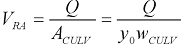(2)
###### Potential Flow Theory

Chang used potential flow principles to derive a velocity adjustment expression to approximate the representative velocity (VRP) that should be used for bridge abutment scour computations.(2) This adjustment compensates for the contraction in flow at the culvert inlet. His expression can be adapted for bottomless culverts, as in equation 3.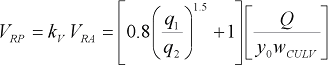(3)

where:

kV
is the ratio of velocity at the culvert toe to the mean velocity in the contracted section.
q1
is unit discharge in the approach section.
q2
is unit discharge in the contracted section.

Equation 3 applies to a simple contraction, where the unit discharge of the approach section, q1, is less than the unit discharge in the contraction section, q2. The ABSCOUR program states that the values of kv should be limited to the range of values between 1.0 and 1.8.(5) If the computed value is less than 1.0, use a value of 1.0; if the computed value is greater than 1.8, use a value of 1.8.

###### Measured Flow Velocity

Since this research produced accurate measurements of the local velocities in the approach section of the culvert, an adjustment was made to the potential flow theory to match the measured flow velocity at the corners of the culvert inlet. This adjustment involved adding a calibration coefficient, C, as given in equation 4.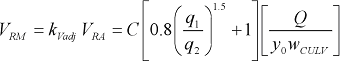(4)
##### Critical Velocity

There are two alternatives for calculating the critical velocity at which incipient sediment motion occurs that are considered in this report: Laursen’s method, and Neill’s method.

###### Laursen’s Critical Velocity Method

Laursen’s equation for the critical velocity is summarized in Appendix C of FHWA Hydraulic Engineering Circular No. 18.(6) The critical velocity, VCL, is calculated by equation 5.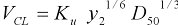(5)

where:

Ku
is 6.19 for SI units, or 11.17 for U.S. customary units.
y2
is equilibrium scour flow depth (m or ft).
D50
is sediment size (m or ft).
###### Neill’s Competent Velocity Method

Neill presented a family of curves for estimating critical velocities for noncohesive sediments for varying flow depths and with grain sizes ranging from 0.3 to 300 mm (0.0117 to 11.7 inches).(7) Neill defined the critical velocity as the flow velocity just competent to move the bed material. Neill used a combination of field data and laboratory data to develop his family of curves. To develop the family of curves, Neill used a critical velocity equation very similar to Laursen’s to estimate the critical velocity for grain sizes greater than about 30 mm (1.17 inches). For a grain size of 0.3 mm (0.0117 inch), Neill assumed that a regime theory equation for stable channels in sand would be appropriate for estimating the critical velocity. (Regime theory equations are design equations developed from field data collected in the stable, fine sediment canals of Pakistan (Mahmood and Shen)).(8) Having defined critical velocities for a grain size of 0.3 mm (0.0117 inch) and for grain sizes greater than 30 mm (1.17 inches), transition curves were hand drawn for grain sizes between 0.3 and 30 mm (0.0117 and 1.17 inches).

Chang transformed the plots of Neill’s curves into a set of equations for computing critical velocity based on the flow depth and the median diameter of the particle.(2) This set is given in equations 6 through 9.
For D50 greater than 0.03 m (0.1 ft), Neill’s critical velocity, VCN, is given in equation 6.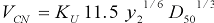(6)

where:

y2
is equilibrium scour flow depth (m or ft).
D50
is sediment size (m or ft).
Ku
is 0.55217 for SI units, or 1.0 for U.S. customary units.

For D50 less than 0.03 m (0.1 ft) but greater than 0.0003 m (0.001 ft), Neill’s critical velocity is given in equation 7.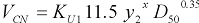(7)

The exponent, x, is calculated using equation 8: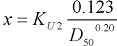(8)

where:

y2
is equilibrium flow depth (m or ft).
D50
is sediment size (m or ft).
KU1
is, for SI units, 0.3048 to the power of 0.65 minus x, or 1.0 for U.S. customary units.
x
is the exponent as calculated in equation 8.
KU2
is 0.788 for SI units, or 1.0 for U.S. customary units.

For D50 less than 0.0003 m (0.001 ft), Neill’s critical velocity is given in equation 9.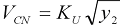(9)

where:

y2
is equilibrium flow depth (m or ft).
D50
is sediment size (m or ft).
Ku
is 0.55217 for SI units, or 1.0 for U.S. customary units.

Chang’s equations are plotted in figure 9. Neill’s competent velocity curves are intended for field conditions with flow depths of 1.5 m (5 ft) or greater. Chang’s equations were extrapolated to flow depths below 0.30 m for these experiments and to curves for flow depths of 0.305 and 0.15 m (1 and 0.5 ft) (see figure 9). Note that the sediment sizes used in the experiments fell into the range described by equations 7 and 8.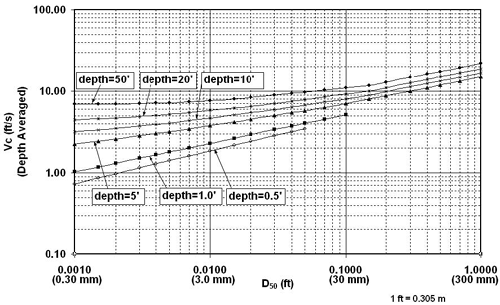Figure 9. Graph. Chang’s approximations to Neill’s competent velocity curves.

##### Adjustment for Spiral Flow at Culvert Toe

This research revealed that the maximum scour depth, ymax (measured at the corners of the culvert), was always greater than the computed equilibrium depth, regardless of which equations for representative velocity and critical velocity were used. Thus, an empirical coefficient kS, similar to an adjustment coefficient, was needed to explain the additional scour depth, as in the following equation: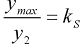(10)

Recalling from the discussion of equation 1 that y2 equals qR divided by VC reveals that kS will be a function of VR and VC, among other things. Our research considered two possibilities for a third independent parameter in the equation for kS: the Froude number at the culvert approach, and a dimensionless ratio including Qblocked and y2. Qblocked is the portion of the approach flow that is to one side of the channel centerline and that is blocked by the embankment as the flow approaches the culvert. Equations 11 and 12 give two different functions for kS.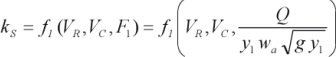(11)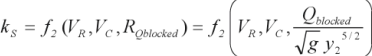(12)

Since there are three different expressions for VR, two different expressions for Vc, and two different expressions for the third independent variable, this research considered 12 different kS values.

##### Adjustment for Pressure Flow at a Submerged Culvert

The maximum scour depth, ymax, measured under submerged conditions, likewise was always greater than the computed equilibrium depth. Thus, an empirical coefficient, kp, was needed to explain the additional scour depth, as in equation 13.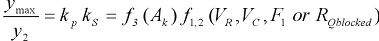(13)

Equation 14 is the equation for Ak.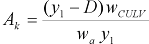(14)

where:

D
is the culvert height at the approach prior to scour.
Ak
is a dimensionless ratio: area of approaching flow directly above the culvert divided by the total area of flow approaching the culvert.

Note that due to the influence of kS, this study will also consider 12 different values for kp. Recall also that yo in equation 1 for pressure flow is equal to the hydraulic grade line at the inlet (HGLo in figure 8). These two different adjustment factors will be derived from experimental data for bottomless culverts in the results section.

#### SCOUR PROTECTION: RIPRAP ANALYSIS

Many researchers have developed critical conditions based on average velocity. Ishbash presented an equation that can be expressed as equation 15.(9)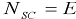(15)

Ishbash described two critical conditions for riprap stability. For loose stones where no movement occurs, NSC is expressed as equation 16.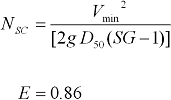(16)

For loose stones allowed to roll until they become “seated,” NSC is expressed as equation 17.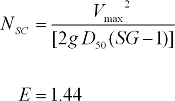(17)

where:

NSC
is computed sediment number for distributed flow.
Vmin
is minimum velocity (ft/s) that will remove the loose stones lying on top of the fill.
Vmax
is maximum velocity (ft/s) that will roll out the stones lying among the others on the slope.
g
is acceleration of gravity (ft/s2).
D50
is diameter of riprap (ft).
SG
is specific gravity of riprap.
E
is the Ishbash constant.

Equation 17 for riprap that will just begin to roll can be written as equation 18. For the culvert experiments, we represented the effective velocity (Veff) in terms of an empirical multiplier (equation 19) and the local bed velocity (equation 20), which is substituted into equation 17 to yield equation 21.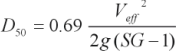(18)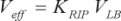(19)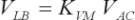(20)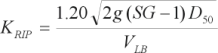(21)

where:

Veff
is effective velocity that accounts for turbulence and vorticity in the mixing zone at the upstream corner of a culvert.
VLB
is local velocity along the bed prior to scour in the vicinity of the upstream corner of a culvert.
VAC
is average velocity in the contracted zone prior to scour in the vicinity of the upstream corner of a culvert.
KRIP
is the coefficient used to size riprap for scour (to be determined in lab experiments).
KVM
is the coefficient relating the local bed velocity in the experiments to the average velocity in the contraction zone (to be determined in lab experiments).
D50
is the diameter of riprap that is expected to be on the verge of failure in the vicinity of the upstream corner of the culvert.

Equations 18 through 21 are dimensionally homogeneous and can be used with either system of units as long as they are consistent.

Previous | Contents | Next